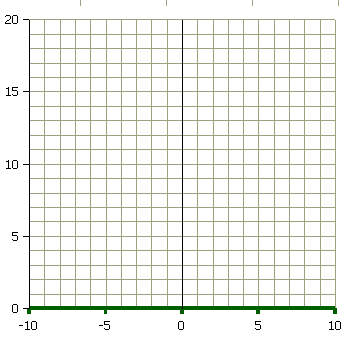### Sum of n numbers: Σ n

Find the following sums:

```1 + 2 =
1 + 2 + 3 =
1 + 2 + 3 + 4 =
1 + 2 + 3 + 4 + 5 =
1 + 2 + 3 + 4 + 5 + 6 =
1 + 2 + 3 + 4 + 5 + 6 + 7 =
1 + 2 + 3 + 4 + 5 + 6 + 7 + 8 =
1 + 2 + 3 + 4 + 5 + 6 + 7 + 8 + 9 = ```
1. _____ What is the sum of the first ten numbers?
2. _____ What is the sum of the first eleven numbers?
3. _____ What is the sum of the first twelve numbers?
4. _____ What is the sum of the first thirteen numbers?
5. _____ Is there a shortcut to finding these sums?
~~~ Class discussion ~~~~

1. _____ What is the sum of the first fourteen numbers?
2. _____ What is the sum of the first fifteen numbers?
3. _____ What is the sum of the first sixteen numbers?
4. _____ What is the sum of the first seventeen numbers?
5. _____ Is there a general formula to finding these sums?
~~~ Class discussion ~~~~

Make a graph of the formula.Homework:

```1 + 3 =
1 + 3 + 5 =
1 + 3 + 5 + 7 =
1 + 3 + 5 + 7 + 9 =
1 + 3 + 3 + 7 + 9 + 11 =
1 + 3 + 3 + 7 + 9 + 11 +13 = ```

Is there a formula for the sum of odd numbers?

What is the formula for the sum of odd numbers?

```2 + 4 =
2 + 4 + 6 =
2 + 4 + 6 + 8 =
2 + 4 + 6 + 8 + 10 =
2 + 4 + 6 + 8 + 10 + 12 =
2 + 4 + 6 + 8 + 10 + 12 +14 = ```

What is the formula for the sum of even numbers?